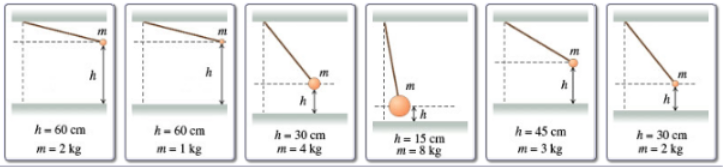# Problem: Rank each pendulum on the basis of its maximum speed.Rank from largest to smallest.

###### FREE Expert Solution

Potential energy:

$\overline{){\mathbf{U}}{\mathbf{=}}{\mathbf{m}}{\mathbf{g}}{\mathbf{h}}}$

Kinetic energy:

At maximum speed, the kinetic energy is maximum. According to the conservation of energy, all the potential energy is converted into kinetic energy.

KEmax = Umax

(1/2)mvmax2 = mghmax

v = (2ghmax)(1/2)

91% (357 ratings)###### Problem Details

Rank each pendulum on the basis of its maximum speed.

Rank from largest to smallest.